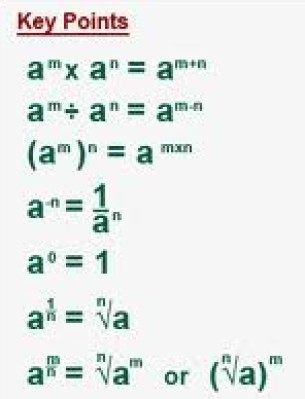List of public pages created with Protopage

# Home

## Plain sticky notes

### Sticky note

Hello!! My name is Madison and this is my protopage!! I hope this helps you with your revision:)

Good Luck!!

## Calendars

### Calendar

• Tue November 6 - UNIT B EXAM!!!!

## Photos

### Remember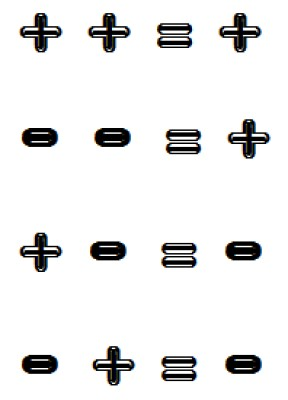### SohCahToa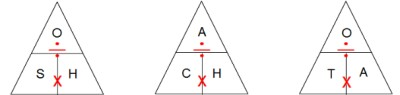# Bookmarks

## Plain sticky notes

### Sticky note

Click 'edit' on the Bookmarks widget to add all of your favorite sites to your page for quick access

# Notes

## Plain sticky notes

### Sticky note

Click this text to change it...

# Unit A

## Plain sticky notes

### Sticky note

In this exam you are allowed to use a calculator!!!

### 1 Pythag

When doing Pythagoras the main rule you need to remeber is -----> This works by following the simple formula, for example if side 'A' is '5' and side 'B' is '7' then you do (5x5)+(7x7) = 25+49 = 64 then work out the square root of 64 = 8 This means that side 'C' is 8 The hypotenuse (side opposite the right angle) is always the longest length so you can check your answer by making sure it is greater than the other lengths.

### 4 Even More Trig

When finding the angle you do 'the inverse operation' this means that you do you formulae to the -1. Sounds confusing... <--- In the example we just used we now know that there are two side measuring 41 and17.33. Lets pretend we dont know that the angle is 25. We know that the side involved are the opposite and the hypotenuse so we use sin. sin(to the power of)-1(17.33/41) = 25 Try it! TIP: to get the sin, cos and tan to the -1 press shift then the operation you must use.

### 2 Trig

Trigonometry is used when trying to find either an angle or a side when only one side or an angle is given. To find a side: You do this by seeing which side you have, in this example ------->> you have the 'hypotenuse' and the 'opposite'is what you are looking for, which means you use the formula 'soh' (below) =sin

### 3 More Trig

This means that you need to compleate the sum 41Sin(25) = 17.33 x=17.33

Remember this ^^

## Photos

### Labeled Triangle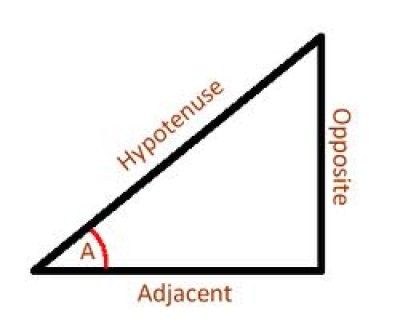### Pythagoras Rule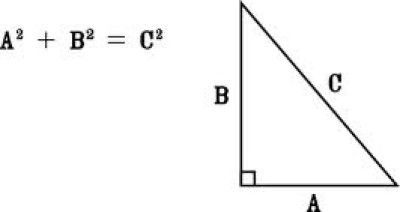### Trigonometry Example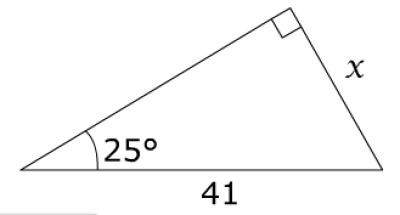### SohCahToa# Unit B

## Plain sticky notes

THIS IS A NON CALCULATOR EXAM!!!!
INDICES!

### 3 What is an INDICE

An indice looks like: 2³ This does not mean 2x3=6 this means 2x2x2 2x2=4x2=8 2³ =8 An indice can be any number... anything to the power of 1 is itself anything to the power of 0 is just 1

### 1 Simultaneous

Working out a sum like: 4x+6y=16 x+2y=5 (x4) 4x+6y=16 4x+8y=20 (-) -2y = -4 y = 2 4x+6(x2)=16 4x+12=16 4x =4 x = 1

### 2 Equations

Working out a sum like: <-- this means when taking away you eliminate the x so you are left with If the 's'igns are the 's'ame 's'ubtract, if they are 'd'ifferent a'dd' You now need to substitute y back into the equation: (pick one preferably the easiest!!) Now work it out! What do you times by 4 to make 4?
Remember these rules and it will be so much easier!

### 4 What Does It Mean?

<----- SO the rules basically mean: any same number to the power of anything when timsing together you just add the indices any same number to the power of anything when dividing you just take away the indices when brackets are involved you times together the two indicies and then whatever your product is do that to the power of your origional number anything to the power of a minus number just take away the minus and work out the sum then put your answer under 1 over anything to the power of 0 is just 1 anything to the power of a fraction when the top number is 1 is the root of the number anything to the power of a fraction is root of the bottom number to the power of the top number

## Photos

### The Indice Rules